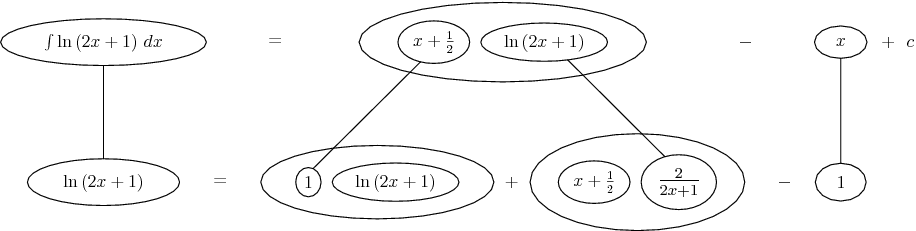# Thread: integration by part

1. ## integration by part

I'm having some trouble with this

$\displaystyle \int{\ln{(2x+1)}dx}$

I set $\displaystyle u = ln(2x+1)$ and $\displaystyle dv = dx$ so that $\displaystyle du=\frac{2}{2x+1}$ and $\displaystyle v = x$.

so after integrating by part once I get:

$\displaystyle x\ln{(2x+1)}-2\int{\frac{x}{2x+1}dx}$

then I tried to integrate by part again with $\displaystyle u=x$ and $\displaystyle dv=(2x+1)^{-1}$

my final answer ends up as $\displaystyle x\ln{(2x+1)}$ but my solution manual tells me differently.

Can anyone tell me what I'm doing wrong?

2.Originally Posted by stevedaveI'm having some trouble with this

$\displaystyle \int{\ln{(2x+1)}dx}$

I set $\displaystyle u = ln(2x+1)$ and $\displaystyle dv = dx$ so that $\displaystyle du=\frac{2}{2x+1}$ and $\displaystyle v = x$.

so after integrating by part once I get:

$\displaystyle x\ln{(2x+1)}-2\int{\frac{x}{2x+1}dx}$

then I tried to integrate by part again with $\displaystyle u=x$ and $\displaystyle dv=(2x+1)^{-1}$

my final answer ends up as $\displaystyle x\ln{(2x+1)}$ but my solution manual tells me differently.

Can anyone tell me what I'm doing wrong?
For the integral $\displaystyle -\int\frac{2x}{2x+1}\,dx$, make the substitution $\displaystyle z=2x+1\implies x=\tfrac{1}{2}\left(z-1\right)$

Thus, $\displaystyle \,dz=2\,dx$.

The integral becomes: $\displaystyle -\int\frac{x\,dz}{z}\implies -\tfrac{1}{2}\int \frac{z-1}{z}\,dz=\tfrac{1}{2}\int\left(\frac{1}{z}-1\right)\,dz$

Can you continue from here?

3.Originally Posted by stevedaveI'm having some trouble with this

$\displaystyle \int{\ln{(2x+1)}dx}$

I set $\displaystyle u = ln(2x+1)$ and $\displaystyle dv = dx$ so that $\displaystyle du=\frac{2}{2x+1}$ and $\displaystyle v = x$.

so after integrating by part once I get:

$\displaystyle x\ln{(2x+1)}-2\int{\frac{x}{2x+1}dx}$

then I tried to integrate by part again with $\displaystyle u=x$ and $\displaystyle dv=(2x+1)^{-1}$

my final answer ends up as $\displaystyle x\ln{(2x+1)}$ but my solution manual tells me differently.

Can anyone tell me what I'm doing wrong?
In the second integral, expand

$\displaystyle \int\frac{x}{2x+1}dx = \int \frac{1}{2} - \frac{1}{2}\frac{1}{2x+1} dx$ and integrate each term separately

BTW $\displaystyle \int \frac{1}{ax+b} dx = \frac{1}{a} \ln| ax + b| + c$

4. $\displaystyle \int \ln(2x+1) \, dx = x\ln(2x+1) - \int \frac{2x}{2x+1} \, dx$

note that $\displaystyle \frac{2x}{2x+1} = \frac{2x+1-1}{2x+1} = 1 - \frac{1}{2x+1}$

$\displaystyle \int \ln(2x+1) \, dx = x\ln(2x+1) - \int 1 - \frac{1}{2x+1} \, dx$

can you finish from here?

5. Thank you everyone. That was very helpful.

6. How about this for a short cut? Notice that one integral of 1 is x + 1/2, and make that the value of v, for dv/dx = 1 ...As usual, straight lines differentiate down / integrate up with respect to x.

Don't integrate - balloontegrate! Balloon Calculus: worked examples from past papers

#### Search Tags

integration